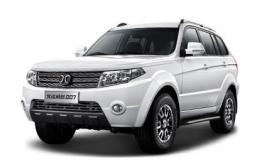# 北汽威旺 北汽威旺007

## 幸福如“7”而至 北汽威旺007邀你塞班双人游

2015年06月16日 13:42 来源：www.chextx.com 超过：6901次关注

继5月8日天猫首发后，北汽威旺007在全国各大区域火热上市，掀起抢购狂潮。现在，这股狂潮伴随着塞班岛的海风再次席卷而来，北汽威旺于6月1日至7月26日推出“北汽威旺007越界上市 双人塞班游等你来拿”有奖购车活动。激爽夏日，威旺007越界驾到，与广大用户一起开拓属于城市越野派的幸福疆土。作为北汽威旺诠释实力质造、技术创新的力作，威旺007以“心致远 行无界”为产品内涵，致力于为崇尚自由又兼顾家庭生活品质的用户带来全新的驾驭体验。威旺007上市以来，得到广大用户如火般的热情支持，如今，北汽威旺再添一把火，诚意回馈，推出“北汽威旺007越界上市 双人塞班游等你来拿”有奖购车活动，豪气送出“注册礼”、“购车礼”及特别惊喜等重重好礼。

第二重“购车礼”——注册报名后，在经销商店内购车并回传发票的用户，有机会获得“购车礼”，活动期间内，抽取10个Apple Watch，以及每周抽取一对塞班双人游幸运大奖，共7对。此外，前70名成功购车并回传发票的用户还可获赠2000元油卡一张，先购先得。多重购车多重惊喜，威旺007让幸福如“7”而至。威旺007为用户开启心灵拓疆之旅的同时，也积极践行在SUV细分市场中的实力拓疆。在群雄割据的SUV市场版图中，威旺007凭借自身过硬的品质和极具优势的性价比频频获赞。

外观硬朗时尚，拓疆路上更拉风。作为北汽威旺旗下首款硬派SUV，威旺007在外观上的表现可谓“刚性”十足，车身采取3U立维设计，线条干练、时尚；采用三条编花式的硕大进气格栅前脸，搭配时下流行的不规则反切式设计的头灯，极富层次感。

对于SUV来说，宽大舒适的乘坐空间是用户的“刚需”，威旺007整车高度、宽度优势十分明显，载物空间也更胜一筹。威旺007的外挂式全尺寸备胎不仅野性十足，更提升了后备厢空间，多达640L的储物空间完全可以满足用户日常和出游的“大”需求。越野性能强悍，拓疆路上更无畏。威旺007采用非承载式车身结构，加上双横臂螺旋弹簧独立前悬架，以及五连杆螺旋弹簧非独立后悬架，提升了转向操纵强度及适应能力。给驾乘人员带来极为顺畅的操控感受及乘坐品质。

威旺007的接近角与离去角分别为30°、29°，再加上16英寸铝合金轮毂以及235/70 R16的专业越野宽胎所赋予的极强抓地力、攀爬力，都使得威旺007拥有较好的越野性能，配合强劲动力，拓疆路上勇往直前、无畏所有挑战。

越野性能的突出，让城市越野派实现拓疆人生、越野无界的梦想。激情夏日，北汽威旺不仅带来了旗下首款全路况SUV，更有“北汽威旺007越界上市 双人塞班游等你来拿”有奖购车活动，为用户带来了满满的幸福感。现在，选择威旺007，开拓幸福疆土，畅游最美海岛，塞班双人游大奖触手可及！

#### 相关文章

0-500 字已有评论 0条 查看评论>>

### 北汽威旺007### 最新资讯

﻿
• 快速找车
• 选择品牌
• 选择品牌
• A  奥迪
• A  阿斯顿·马丁
• A  阿尔法·罗密欧
• B  宝沃
• B  布加迪
• B  巴博斯
• B  保时捷
• B  宾利
• B  奔驰
• B  宝马
• B  本田
• B  别克
• B  标致
• B  比亚迪
• B  宝骏
• B  北汽制造
• B  北汽新能源
• B  北汽幻速
• B  北汽威旺
• B  北京汽车
• B  奔腾
• B  北汽绅宝
• C  长安
• C  长安商用
• C  长城
• C  昌河
• D  大众
• D  道奇
• D  DS
• D  东南
• D  东风风神
• D  东风风行
• D  东风小康
• D  东风风度
• D  东风
• F  福特
• F  丰田
• F  菲亚特
• F  法拉利
• F  福田
• F  福迪
• F  福汽启腾
• G  观致
• G  广汽传祺
• G  广汽吉奥
• G  GMC
• H  红旗
• H  汉腾汽车
• H  哈弗
• H  哈飞
• H  海格
• H  海马
• H  华颂
• H  黄海
• H  华泰
• H  恒天
• J  吉利汽车
• J  捷豹
• J  Jeep
• J  江淮
• J  江铃
• J  金杯
• J  九龙
• J  金旅
• K  凯翼
• K  凯迪拉克
• K  克莱斯勒
• K  科尼塞克
• K  卡威
• K  开瑞
• L  路虎
• L  林肯
• L  劳斯莱斯
• L  兰博基尼
• L  雷克萨斯
• L  铃木
• L  雷诺
• L  理念
• L  力帆
• L  莲花汽车
• L  猎豹
• L  路特斯
• L  陆风
• M  马自达
• M  MG
• M  MINI
• M  玛莎拉蒂
• M  摩根
• M  迈凯轮
• N  纳智捷
• O  欧宝
• O  讴歌
• O  欧朗
• Q  奇瑞
• Q  起亚
• Q  启辰
• R  日产
• R  荣威
• R  瑞麒
• S  三菱
• S  斯威汽车
• S  萨博
• S  smart
• S  斯柯达
• S  斯巴鲁
• S  思铭
• S  双龙
• S  上汽大通
• S  双环
• T  特斯拉
• T  腾势
• W  沃尔沃
• W  五菱汽车
• W  五十铃
• W  威兹曼
• W  威麟
• X  现代
• X  雪佛兰
• X  雪铁龙
• X  西雅特
• Y  一汽
• Y  英菲尼迪
• Y  英致
• Y  依维柯
• Y  野马汽车
• Y  永源
• Z  众泰
• Z  中华
• Z  中兴
• Z  知豆
• 选择车系
• 选择车系
• 车型对比
• 选择品牌
• 选择品牌
• A  奥迪
• A  阿斯顿·马丁
• A  阿尔法·罗密欧
• B  宝沃
• B  布加迪
• B  巴博斯
• B  保时捷
• B  宾利
• B  奔驰
• B  宝马
• B  本田
• B  别克
• B  标致
• B  比亚迪
• B  宝骏
• B  北汽制造
• B  北汽新能源
• B  北汽幻速
• B  北汽威旺
• B  北京汽车
• B  奔腾
• B  北汽绅宝
• C  长安
• C  长安商用
• C  长城
• C  昌河
• D  大众
• D  道奇
• D  DS
• D  东南
• D  东风风神
• D  东风风行
• D  东风小康
• D  东风风度
• D  东风
• F  福特
• F  丰田
• F  菲亚特
• F  法拉利
• F  福田
• F  福迪
• F  福汽启腾
• G  观致
• G  广汽传祺
• G  广汽吉奥
• G  GMC
• H  红旗
• H  汉腾汽车
• H  哈弗
• H  哈飞
• H  海格
• H  海马
• H  华颂
• H  黄海
• H  华泰
• H  恒天
• J  吉利汽车
• J  捷豹
• J  Jeep
• J  江淮
• J  江铃
• J  金杯
• J  九龙
• J  金旅
• K  凯翼
• K  凯迪拉克
• K  克莱斯勒
• K  科尼塞克
• K  卡威
• K  开瑞
• L  路虎
• L  林肯
• L  劳斯莱斯
• L  兰博基尼
• L  雷克萨斯
• L  铃木
• L  雷诺
• L  理念
• L  力帆
• L  莲花汽车
• L  猎豹
• L  路特斯
• L  陆风
• M  马自达
• M  MG
• M  MINI
• M  玛莎拉蒂
• M  摩根
• M  迈凯轮
• N  纳智捷
• O  欧宝
• O  讴歌
• O  欧朗
• Q  奇瑞
• Q  起亚
• Q  启辰
• R  日产
• R  荣威
• R  瑞麒
• S  三菱
• S  斯威汽车
• S  萨博
• S  smart
• S  斯柯达
• S  斯巴鲁
• S  思铭
• S  双龙
• S  上汽大通
• S  双环
• T  特斯拉
• T  腾势
• W  沃尔沃
• W  五菱汽车
• W  五十铃
• W  威兹曼
• W  威麟
• X  现代
• X  雪佛兰
• X  雪铁龙
• X  西雅特
• Y  一汽
• Y  英菲尼迪
• Y  英致
• Y  依维柯
• Y  野马汽车
• Y  永源
• Z  众泰
• Z  中华
• Z  中兴
• Z  知豆
• 选择车系
• 选择车系
• 选择车型
• 选择车型
• 意见反馈